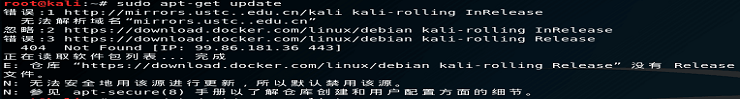# [Hacking walkthrough] THM: ScriptingHowdy, welcome to another tryhackme CTF walkthrough. This is my personal favorite room because it involves scripting and ciphering. As you know, I’m a die-hard fan for forensic and programming :p . For your information, There are a total of 3 stages for this challenge where the first stage is decoding base64, port capture on stage 2 and finally, the hardest stage (perhaps), decrypting the AES-GCM block cipher. Let’s get started

You are required to write a script to decode the base64 for 50 times. Bash and python, both works for you but I prefer python. Copy the following script and execute along with the file.

``````#b64.py
import base64
import sys

with open(sys.argv,'r') as my_file:

for i in range (0,50):
data = base64.b64decode(data)

print(data)
my_file.close()``````
``\$ python b64.py b64.txt``

Simple huh. Well, the best is yet to come.

## Task 2: Capture the port

This is a fun challenge, to be honest. Your task is to follow the port and reveal the mathematic operation. You need to have basic knowledge on python socket, If you need a full guide on the socket python, I highly recommend this article. For me, I am a lazy person, well no doubt. So, I letting the python do the mathematic for me.

These are the flow of my code
1: Initialize port 1337 as staring port (Why? Read the page, duh)
2. Initialize the socket
3. Send an HTTP GET request
5. Process the response (trim, replace, split)
6. Perform arithmetic
7. Repeat step 2 to 6 until STOP response

To avoid the script getting terminate due to connection refused error, I used ‘try’ and ‘except’ with ‘pass’. Alright, the code is right here (I know you need it)

``````import socket
import sys
import time

host=sys.argv
port = 1337
number = 0

while 1:
try:
s = socket.socket()
s.connect((host,port))
if (port == 9765):
break
old_port = port
request = "GET / HTTP/1.1\r\nHost:%s\r\n\r\n" % host
s.send(request.encode())
response = s.recv(4096)
http_response = repr(response)
http_trim = http_response[167:]
http_trim = http_trim.replace('\'','')
data_list = list(http_trim.split(" "))
port = int(data_list)
print('Operation: '+data_list+', number: '+ data_list+', next port: '+ data_list)
if(port != old_port):
number += float(data_list)
elif(data_list == 'minus'):
number -= float(data_list)
elif(data_list == 'multiply'):
number *= float(data_list)
elif(data_list == 'divide'):
number /= float(data_list)
s.close()
except:
s.close()
pass

print(number)``````
``\$ python pn.py <Machine IP>``

For your information, the port on the webserver is recycling (there are a total of 35 ports). Which mean the port changing sequence is always fixed. If you miss a port because of the poor connection, don’t worry, you just need to wait for another round (140-second per round). Sit back and relax until you get the number. Just let me know if you have a better script ?

Here you go.

Here comes my favorite part, the block cipher. Oh yes!!!!!! Block cipher FTW. (cough) sorry. For this task, you are required to decrypt the AES-GCM mode block cipher. But before that, you need to make a socket connection (like the previous task) with the UDP server.

Alright, this is the flow of my code
2) Send payload ‘ready’ to reveal Keys, IV, and checksum for the flag
3) send payload ‘final’ TWICE to receive encrypted flag followed by the tag
4) Decrypt the flag
5) Check the plaintext with checksum. If checksum not match, repeat step 4
6) Print the plaintext if checksum match

``````import socket
import sys
import hashlib

from cryptography.hazmat.backends import default_backend
from cryptography.hazmat.primitives.ciphers import (
Cipher, algorithms, modes
)

host = sys.argv
port = 4000

def AES_GCM_decrypt(key, iv, ciphertext, tag):
associated_data = ''
decryptor = Cipher(algorithms.AES(key), modes.GCM(iv,tag), backend=default_backend()).decryptor()
return decryptor.update(ciphertext) + decryptor.finalize()

def SHA256_hash(hash_string):
sha_signature = hashlib.sha256(hash_string.encode()).hexdigest()
return sha_signature

s = socket.socket(socket.AF_INET, socket.SOCK_DGRAM)
s.connect((host,port))

s.send(b'hello\n')
data = s.recv(2048)
print(data)

hex_checksum = checksum.encode('hex')
print("checksum in hex: "+hex_checksum)

while 1:
s.send(b'final\n')
flag = s.recv(2048)

s.send(b'final\n')
tag = s.recv(2048)

key = b'thisisaverysecretkeyl337'
iv = b'secureivl337'
tag = bytes(tag)
ciphertext = bytes(flag)
plaintext = AES_GCM_decrypt(key, iv, ciphertext, tag)

hash_string = SHA256_hash(plaintext)
if(hash_string == hex_checksum):
print('flag is: '+plaintext)
break

s.close()``````

Not that hard huh.